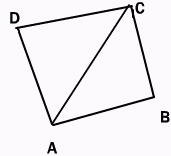Email us to get an instant 20% discount on highly effective K-12 Math & English kwizNET Programs!

#### Online Quiz (WorksheetABCD)

Questions Per Quiz = 2 4 6 8 10

### High School Mathematics - 2 Area of Quadrilateral

 The area of quadrilateral can be found by splitting it into two triangles and ading up their areas. The area of the quadrilateral whose vertices taken in order are A(x1, y1), B(x2, y2), C(x3, y3), D(x4, y4).Write down the coordinates as shownArea of Quad ABCD = Area of triangle ABC + Area of triangle ACD = 1/2{(x1y2)-(x2y1+(x2y3-x3y2(x3y1-x1y3)} + 1/2{(x1y3-x3y1)+x3y4-x4y3)+ (x4y1-x1y4} =1/2[(x1y2-x2y1)+(x2y3-x3y2)+(x3y4-x4y3)+(x4y1-x1y4)] Example: Find the area of the quadrilateral whose vertices are (3.4), (0,5),(2,-1) and (3,-2).The required area is 1/2[3x5-0x4+0x(-1)-5x2+2x(-2)-(-1)(3)+3x4-(-2)(3)] Answer: 11 sq.units Directions: Solve the following.